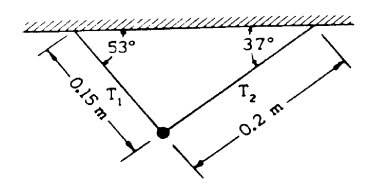# Finding Tension with angles

## Homework Statement

A ball of weight 5 newtons is suspended by two strings as shown above. Determine the magnitude of each of the forces, T1 and T2. (Note: sin 37° = 0.6; cos37° = 0.8)T = mg

## The Attempt at a Solution

I couldn't go anywhere because I need a formula that uses sin or cos, and I can't remember any right now. Can anyone supply me with the equations and a start? And I'll attempt at the solution with that. Please.

Last edited:

Would this work? T x cos θ1 + T x cos θ2 = mg, where θ1 and θ2 are the angles the two sides.

berkeman
Mentor

## Homework Statement

A ball of weight 5 newtons is suspended by two strings as shown above. Determine the magnitude of each of the forces, T1 and T2. (Note: sin 37° = 0.6; cos37° = 0.8)

http://bb.mivu.org/courses/1/M-APhB1-S112-01/assessment/685f98ac2d604bddbf30b5bb28f9f01b/Q5.jpg

T = mg

## The Attempt at a Solution

I couldn't go anywhere because I need a formula that uses sin or cos, and I can't remember any right now. Can anyone supply me with the equations and a start? And I'll attempt at the solution with that. Please.

Would this work? T x cos θ1 + T x cos θ2 = mg, where θ1 and θ2 are the angles the two sides.

Oops, there I edited the first post and it should be there now.

Also, after I posted that second post, I realized that nowhere in there it had any variables for the length of the string, which likely play a part in here. So I think I'm not supposed to use that equation.

berkeman
Mentor
Oops, there I edited the first post and it should be there now.

Also, after I posted that second post, I realized that nowhere in there it had any variables for the length of the string, which likely play a part in here. So I think I'm not supposed to use that equation.

On these problems, always start by drawing a Free Body Diagram (FBD) of the object (the mass in this case). Show the forces on the object, including the force down due to gravity. Then write the two equations to sum all of the forces in the x and y directions to zero (since the object is not moving). then solve away!

I have one drawn right in front of me right now, but I just don't know what formula to use, or how to find ALL of the forces in both axis.

berkeman
Mentor
I have one drawn right in front of me right now, but I just don't know what formula to use, or how to find ALL of the forces in both axis.

How many forces act in the horizontal direction? What are they? Label forces in the +x direction positive, and forces in the -x direction negative.

How many forces act in the vertical direction? What are they? Forces up are positive, and forces down are negative.

Since the object is not accelerating, you know that the sum of the forces in the x direction needs to equal zero. Same thing for the y direction. Those are the two equations you write and solve for the tension forces.

In the x direction T1 - T2 = 0?

And in the y direction. T1 + T2 = mg?

So i'll have to use Trig to find the numbers for each axis, but the only number I have is length, how would that help me?

berkeman
Mentor
In the x direction T1 - T2 = 0?

And in the y direction. T1 + T2 = mg?

So i'll have to use Trig to find the numbers for each axis, but the only number I have is length, how would that help me?

That is much closer. Good.

But you need to use the "components" of the tensions in the x and y directions, and that is where the sin and cos functions come in. Those are used to resolve vectors (like the tension forces applied at angles) into their x and y components.

See the "Intro to Vector Mathematics" link at the bottom of this FBD tutorial, for example: